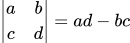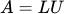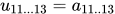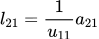# Calculating Matrix Determinants in C

I’ve just finished a research project focused around creating a general classifier for a large data-set. It deals with time series data, and as such must split this time series data into segments using change point detection. In one of the cost functions I was using, it needs to calculate the determinant of a matrix. I’ve hashed together some matrix manipulation code before for Curve Fitting on an Arduino, but this was just to solve a problem and wasn’t particularly thought about. Now I’ve had some time I wanted to do this properly.

## What is a matrix determinant?

A determinant of a matrix can mean different things in different contexts. When working with a covariance matrix, it measures the “Differential Entropy” of the system. It’s used in Cramer’s rule to do least squares regression too.

## How is it calculated?

It can only be calculated with square matrices, and for a 2×2 matrix is calculated byWhen the matrices get any bigger than 2×2 however, then it gets a little more tricky. There’s a recursive method that can be used where you divide the matrix into sub-matrices (called minors), formally this is called Laplace Expansion and is performed in O(n!) time, which is huge! The best that determinants can be calculated in is O(n^2.373), which is still pretty bad, but that’s using a Fast Matrix Multiplication algorithm. We can do something in the middle, how does O(n^3) sound? This is using a method called LU Decomposition, which, oddly enough is also really useful for solving systems of linear equations. Let’s dive into the code!

## Arrays in C

This subject has been done to death, so I’m not going to dive into this. Instead I’ve chosen to use a flat array here for simplicity. Mainly because the memory will be contiguous (all in the same place) and we only have to deal with one pointer, a `double*`.

## Defining a matrix

Ok, so first we need to structure our matrix so we know things like its number of rows and columns. Let’s use a struct.

``````typedef struct Matrix{
size_t rows;
size_t cols;
double* matrix;
} Matrix;``````

Since we’re working with a flat list, we need a simple way to access the elements in it using row, col notation.

``````double m_get(Matrix* mat, size_t i, size_t j){
return mat->matrix[i*mat->cols + j];
}``````

We assume the flat list has each row one after the other, so if we want row 2, column 3 in a 3 column matrix, we need to access the 1*3 + 2th index of the flat array. Now we can access our matrix with `m_get(mat, 0, 0)`.

But how do we create a matrix? We could either make a function that `malloc`‘s some memory for us, or create it ourselves in the scope of `main`. I’m not a fan of `malloc`‘ing until I have to. so let’s create it manually.

``````double data = {1, 2, 3, 4, 5, 6, 7, 8, 9};
Matrix mat = {rows: 3, cols: 3, matrix: &data};``````

Nice! Try to access a few of the elements with `m_get` and print them with `printf`.

## LU Decomposition

To perform LU Decomposition we need to split a given matrix, A into lower and upper matrices, L and U such thatExpanded for a 3×3 matrix (using this as a reference)If we work back from the last matrix, we can see how to calculate each value for L and U.Then for the next rowNOTE: This is a work in progress, but the full code can be found here